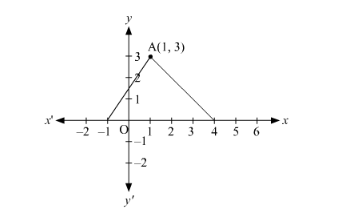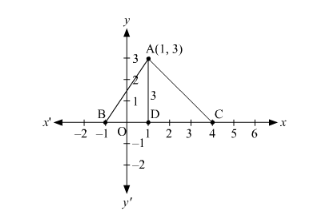# In Fig. 14.46, the area of ΔABC (in square units) is

Question:

In Fig. 14.46, the area of ΔABC (in square units) is                                                                                                                     [CBSE 2013]

(a) 15

(b) 10

(c) $7.5$

(d) $2.5$Solution:The coordinates of A are (1, 3).

∴ Distance of A from the x-axis, AD = y-coordinate of A = 3 units

The number of units between B and C on the x-axis are 5.

∴ BC = 5 units

Now,

Area of $\triangle \mathrm{ABC}=\frac{1}{2} \times \mathrm{BC} \times \mathrm{AD}=\frac{1}{2} \times 5 \times 3=\frac{15}{2}=7.5$ square units

Thus, the area of ∆ABC is 7.5 square units.

Hence, the correct answer is option C.